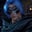Related Tags

julia

# How to get the inverse of a matrix JuliaGutha Vamsi Krishna

## Overview

In Julia, matrices can store heterogeneous elements. It dynamically decides the type of the value. In this shot, we will learn how to get the inverse of a matrix using Julia.

The inverse of a matrix is another matrix which, upon multiplication with the given matrix, gives the identity matrix.

For instance, if $A$ is the given matrix, then $A * A^{-1} = I$.

We can get the inverse of the matrix in Julia using the inv() function.

### Syntax

inv(matrix)

### Parameters and return value

It accepts a matrix as a parameter and returns the inverse matrix of it.

### Example

Let us take a look at an example.

#give matrix
A = [1 -1; 0 2]

#get inverse of matrix
display(inv(A))

### Explanation

In the code snippet above:

• Line 2: We declare and initialize matrix A.
• Line 5: We use the inv() function to get the inverse of the matrix A and display the returned inverse matrix of A.

RELATED TAGS

julia

CONTRIBUTORGutha Vamsi Krishna
RELATED COURSES

View all Courses

Keep Exploring

Learn in-demand tech skills in half the time